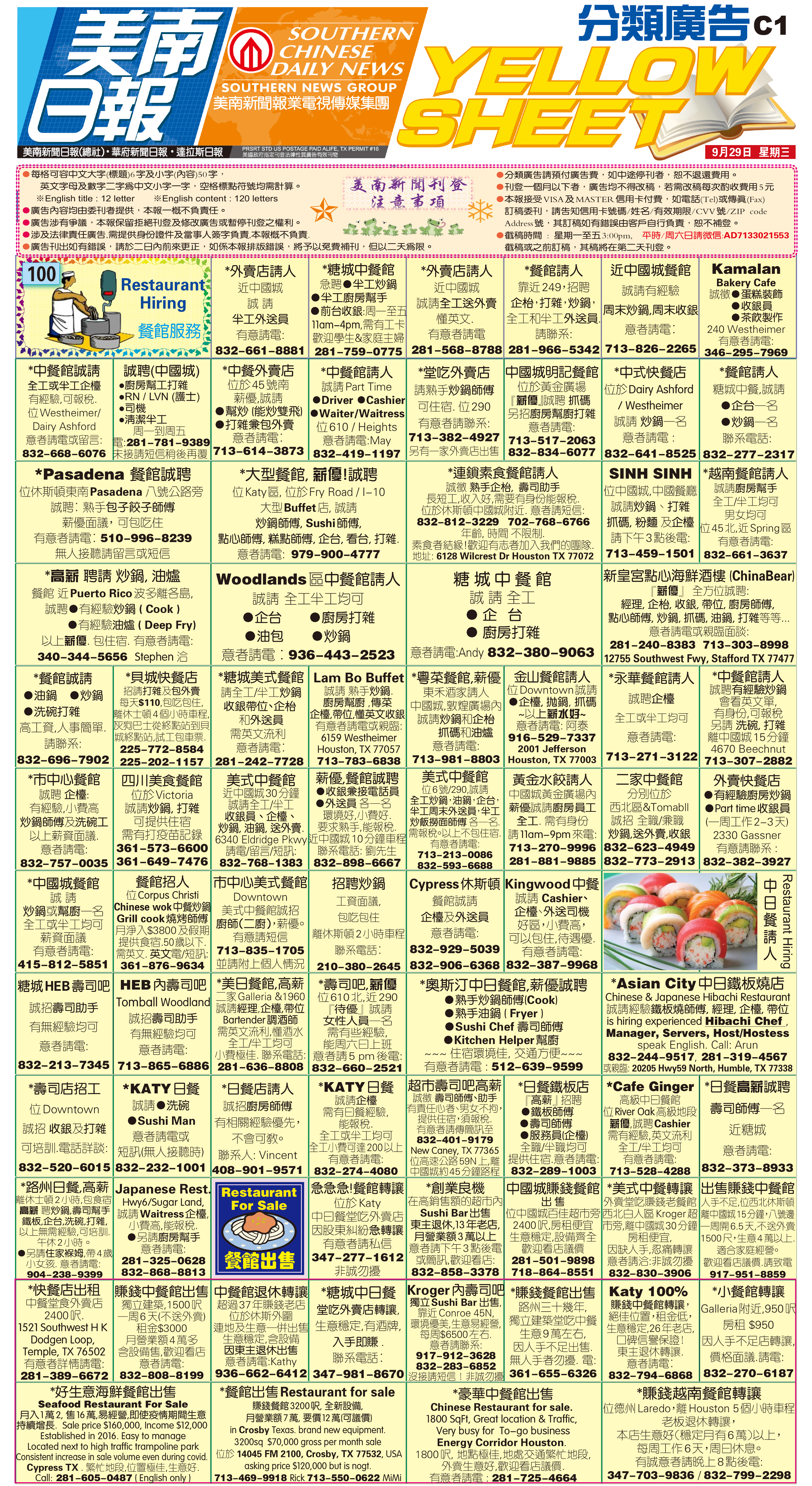211009 Epaper

 A Section B SectionC Section D Section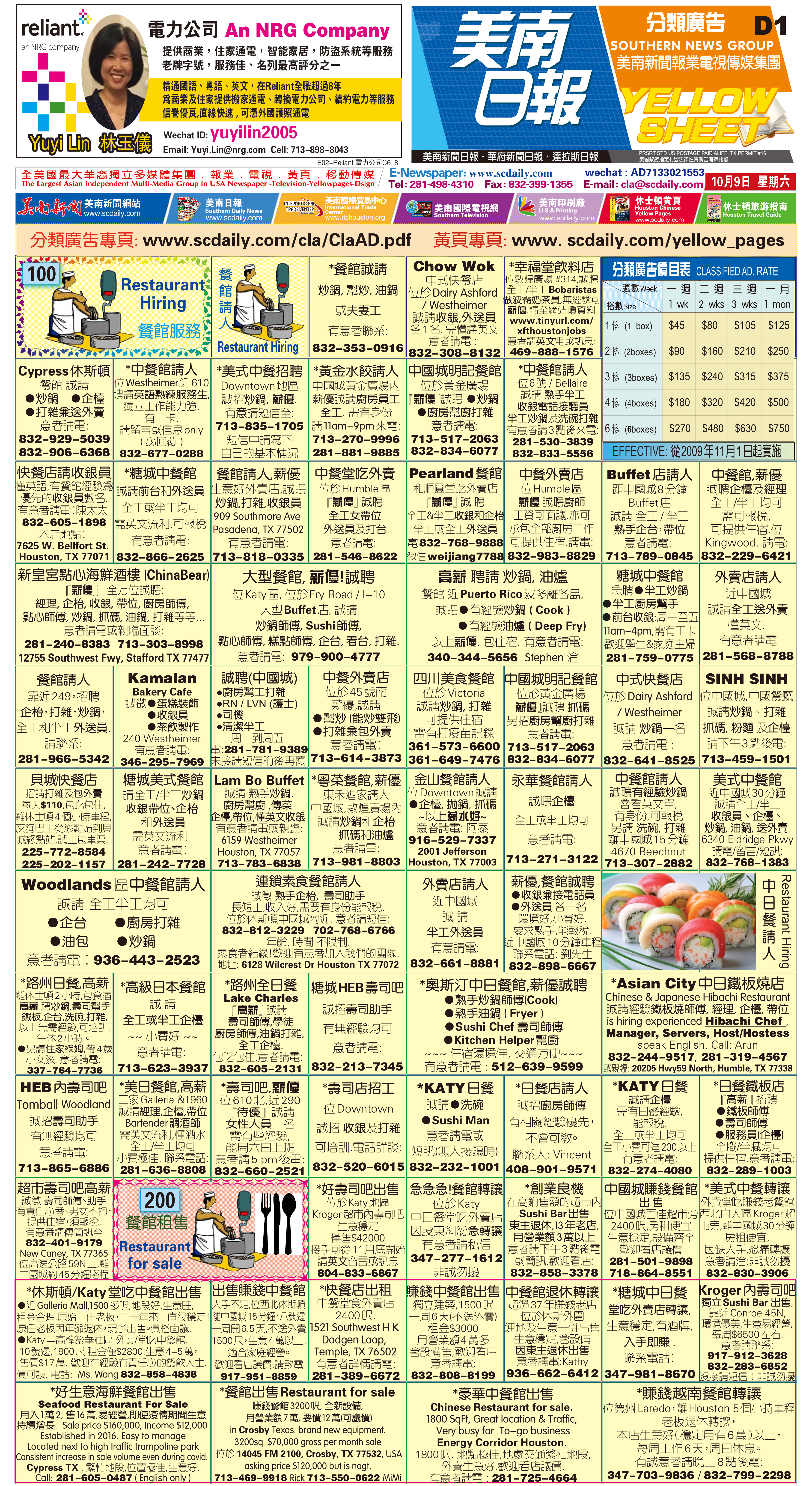211008 Epaper

 A Section B SectionC Section D Section211007 Epaper

 A Section B SectionC Section D Section211006 Epaper

 A Section B SectionC Section D Section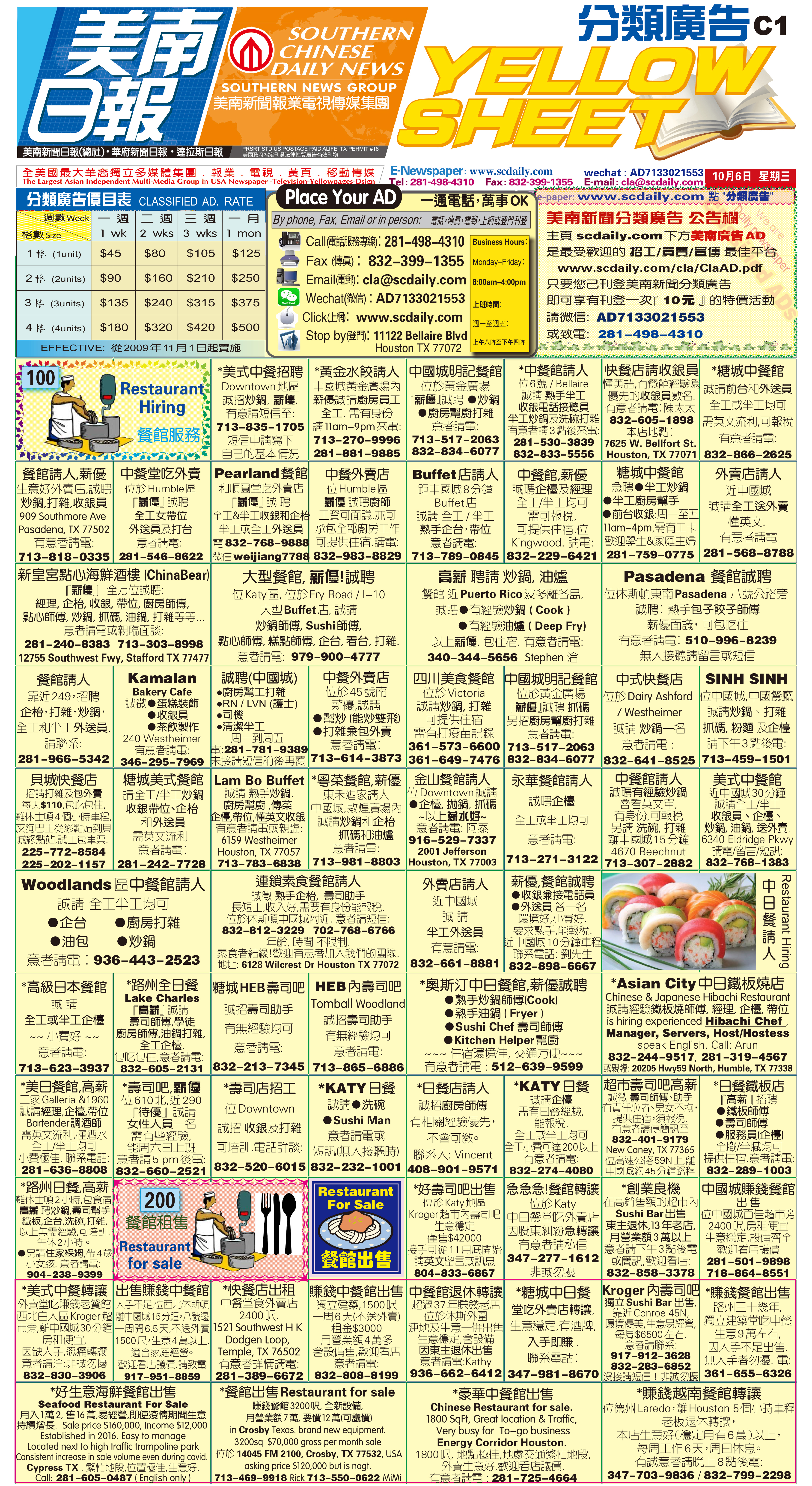211005 Epaper

 A Section B SectionC Section D Section211004 Epaper

 A Section B SectionC Section D Section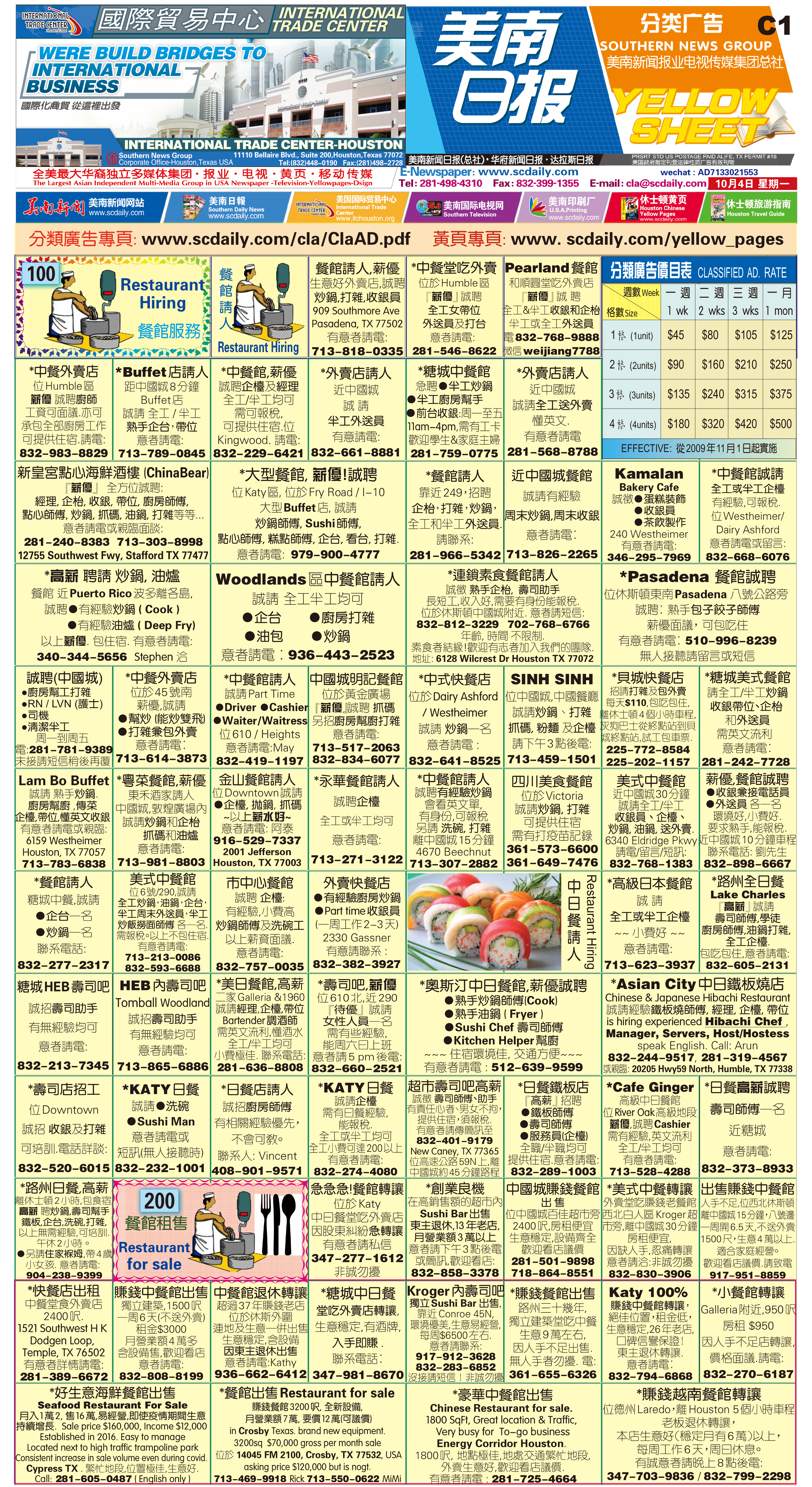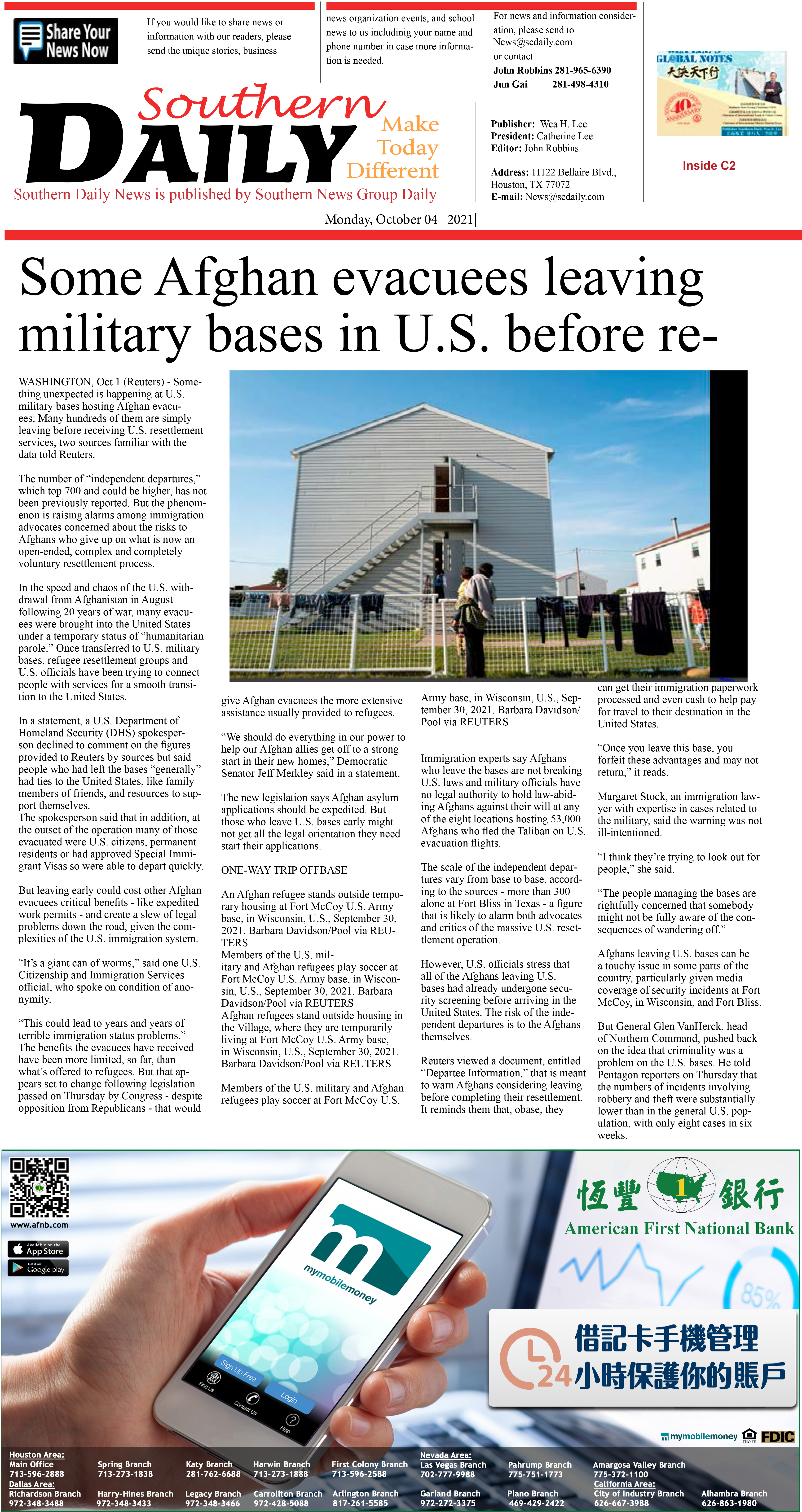211003 Epaper

 A Section B SectionC Section D Section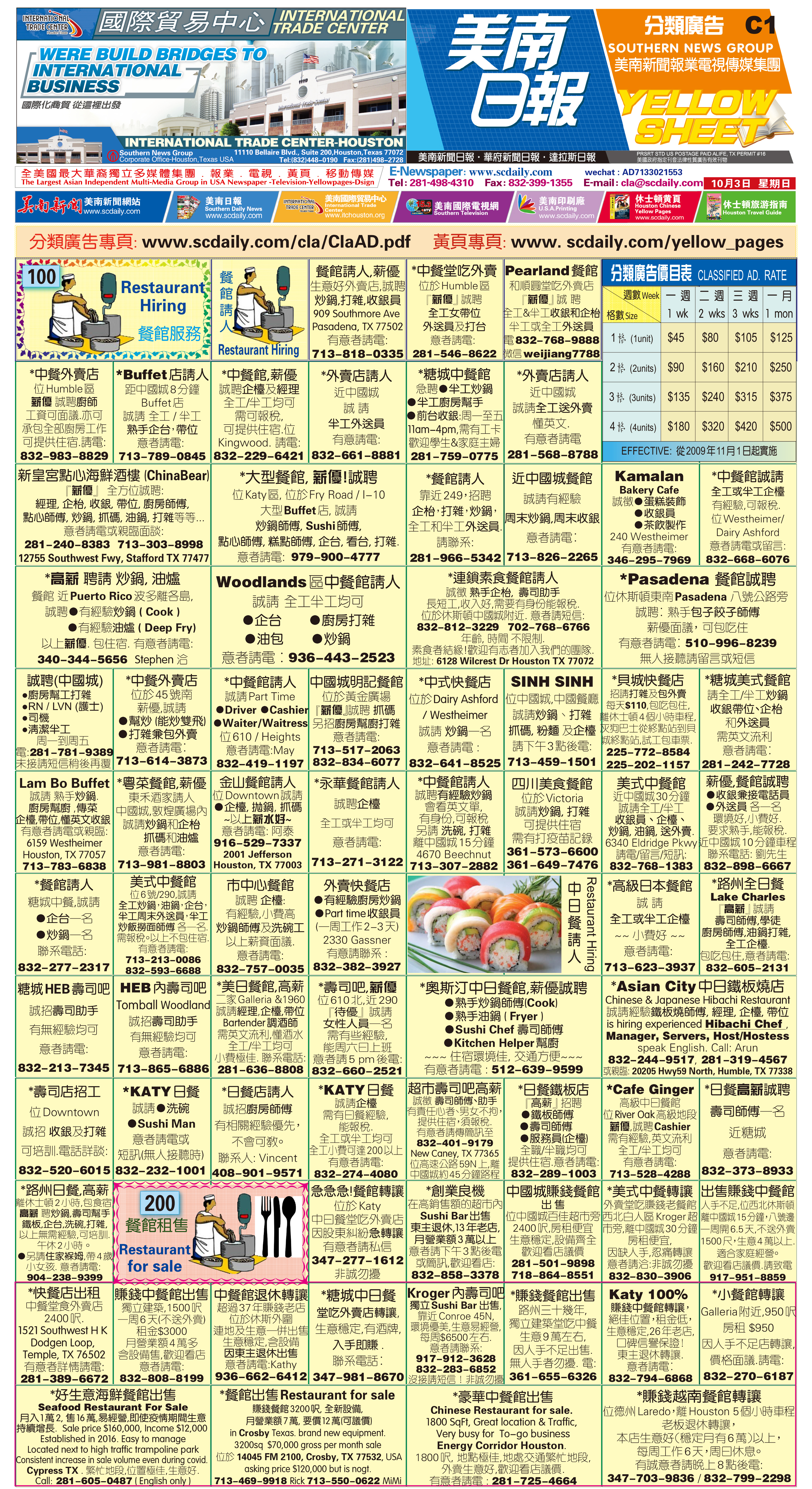211002 Epaper

 A Section B SectionC Section D Section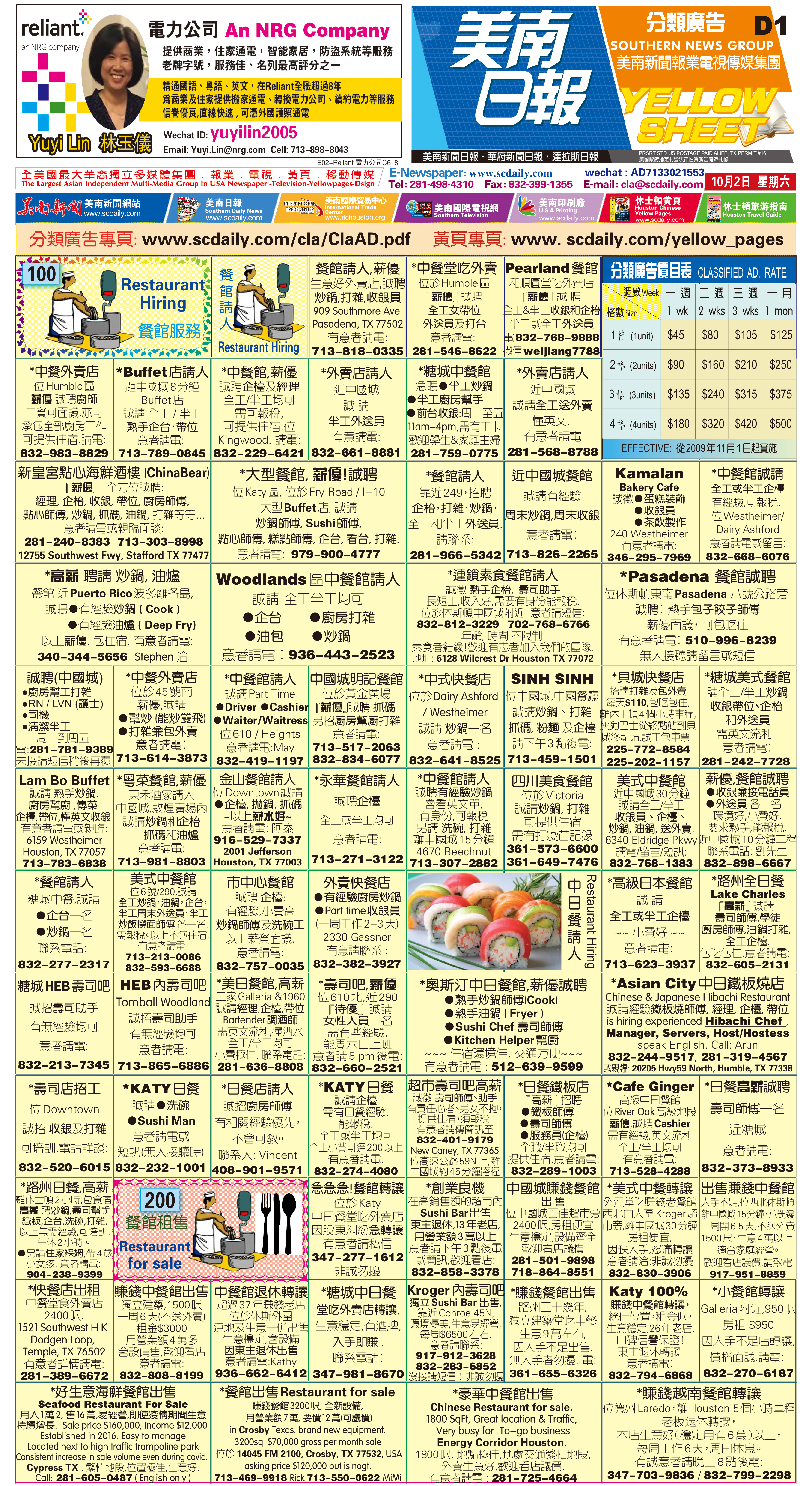210930 Epaper

 A Section B SectionC Section D Section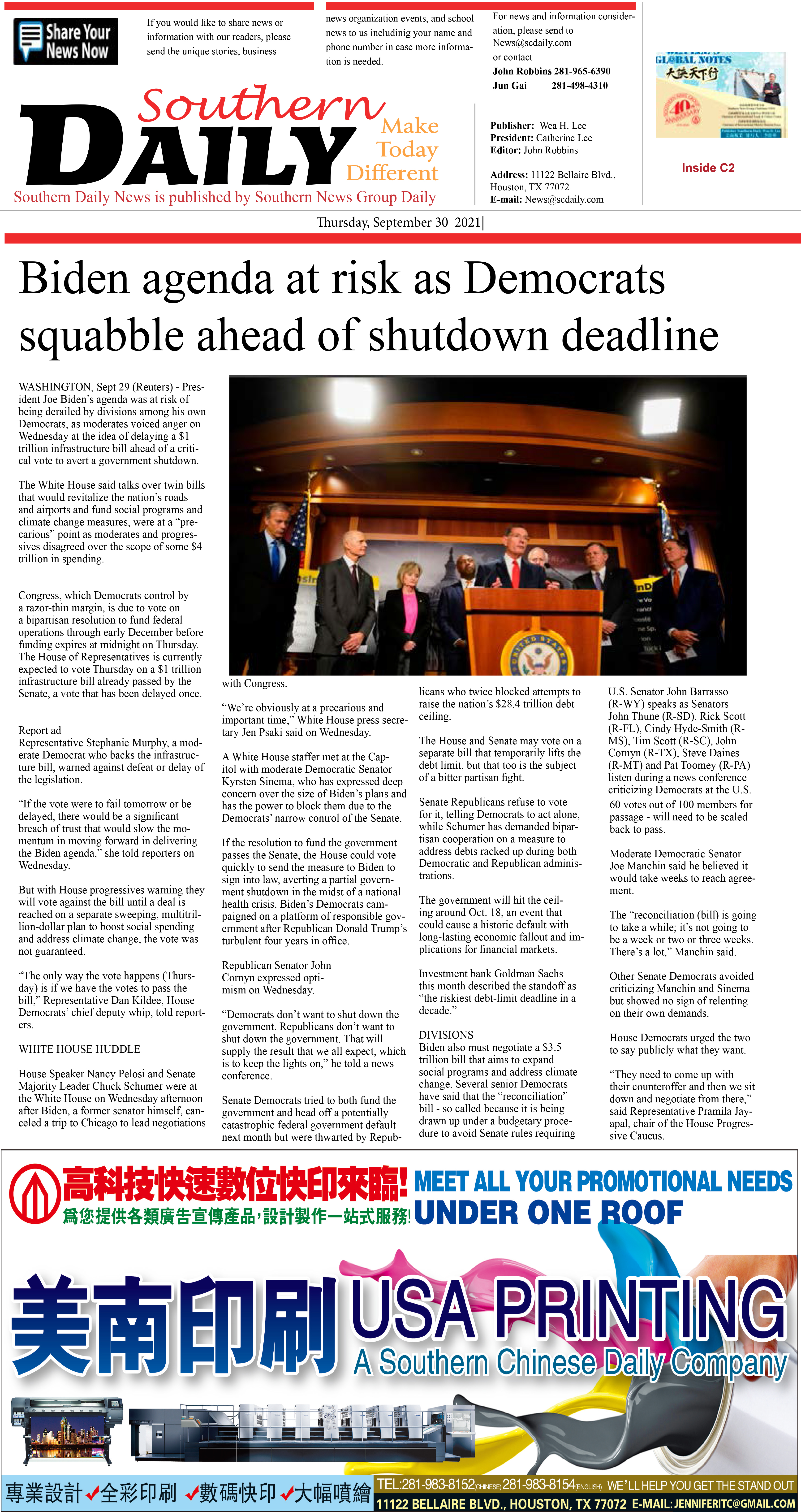211001 Epaper

 A Section B SectionC Section D Section210929 Epaper

 A Section B SectionC Section D Section